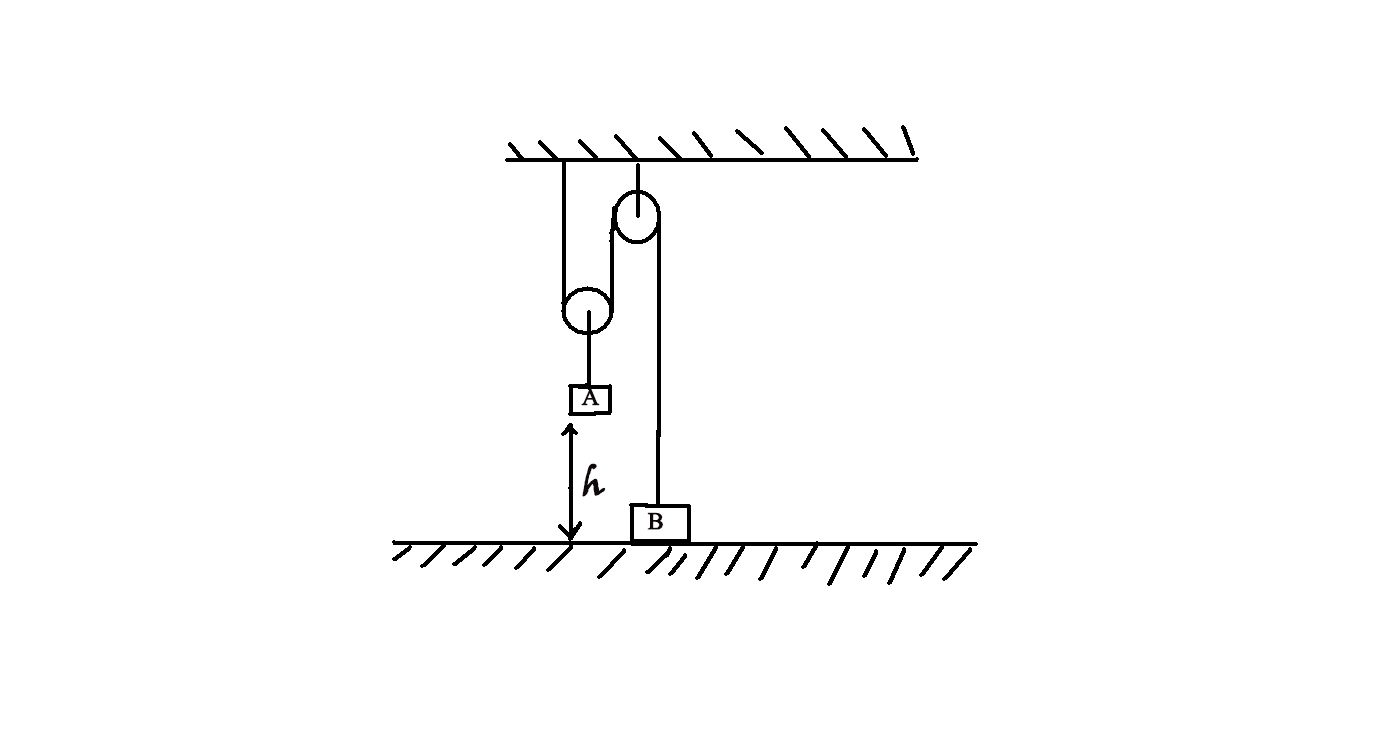# Arrangement in motionIn the arrangement shown in figure, the mass of the body A is 4 times that of body B. The height h=20 cm . At a certain instant, the body B is released and the system is set in motion. What is the maximum height (in cm), the body B will go up? Assume enough space above B and A sticks to the ground. ($g= 10ms^{-2}$)

×